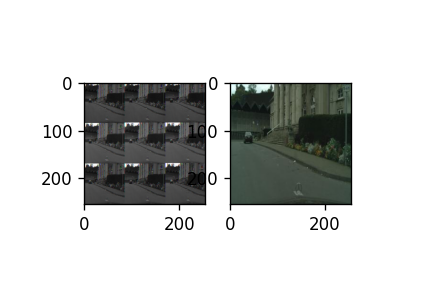# Torchvision output issue

The output image of torchvision.transforms return a batch of same images turned grey, even though it suppose to turn it into tensor. I attach the image of the generated output(left) and the reference of the image taken from dataset(right). I was trying to look on the forums but I havent found anything also I tested it on google colab and jupyter notebook with the same result. Is that the way how every image in Pytorch is transformed?``````class Cityscapes(Dataset):
def __init__(self, path, transform = None):
self.root_dir = path
self.images = self.root_dir + r"train/"
self.labels = self.root_dir + r"val/"
print(self.labels, self.images)
self.transform = transform

def __len__(self):
return len(self.labels)

def __getitem__(self, index):
image = np.array(Image.open(self.images + str(index+1) + '.jpg').convert('RGB'))
label = np.array(Image.open(self.labels + str(index+1) + '.jpg')
temp = image
if self.transform is not None:
image = self.transform(image)

return image, temp
``````
``````
t = torchvision.transforms.Compose([
torchvision.transforms.ToTensor()
``````

The left image is interleaved, which is usually caused by a wrong usage of `view` or `reshape` to swap the dimensions of an array or tensor instead of using `permute`.
Could you check your code, if this could also be the case here?

Unfortunetly the image is not reshaped, or permuted, it becomes interleaved after applying torchvision.transforms.toTensor.

Could you upload the input image here and post the transformation so that we could try to debug it, please?

Of course.Before I tried also different transformation like rotation with the same result.

``````t = torchvision.transforms.Compose([
torchvision.transforms.ToTensor()
])
``````

Thanks! The transformation works as intended and this code properly visualizes the normalized image:

``````img = PIL.Image.open('tmp02.jpeg')

transform = torchvision.transforms.Compose([
torchvision.transforms.ToTensor()
])

out = transform(img)
plt.imshow(out.permute(1, 2, 0).numpy())
``````Feel free to post an executable code snippet, which would reproduce this issue.

Cheers, it works I put it in a return of dataset function, but still is there an explaination why the image turns into interleaved?

The image won’t be interleaved automatically, and since I’m not able to reproduce the issue you would have to check for `view` or `reshape` operations in your code, which would interleave the input.